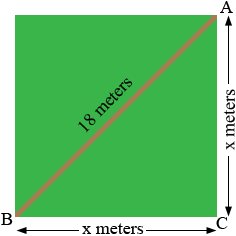SEARCH HOMEMath Central Quandaries & QueriesQuestion from felicia, a parent: a diagonal walkway through a park is 18 meters long. If the park is a square, how long is one of its sides to the nearest tenth of a meter?Felicia,

Since the park is a square the lengths of the 4 sides are identical. Suppose the length of a side is x meters.Triangle ABC is a right triangle. What does Pythagoras tell you?

PennyMath Central is supported by the University of Regina and The Pacific Institute for the Mathematical Sciences.